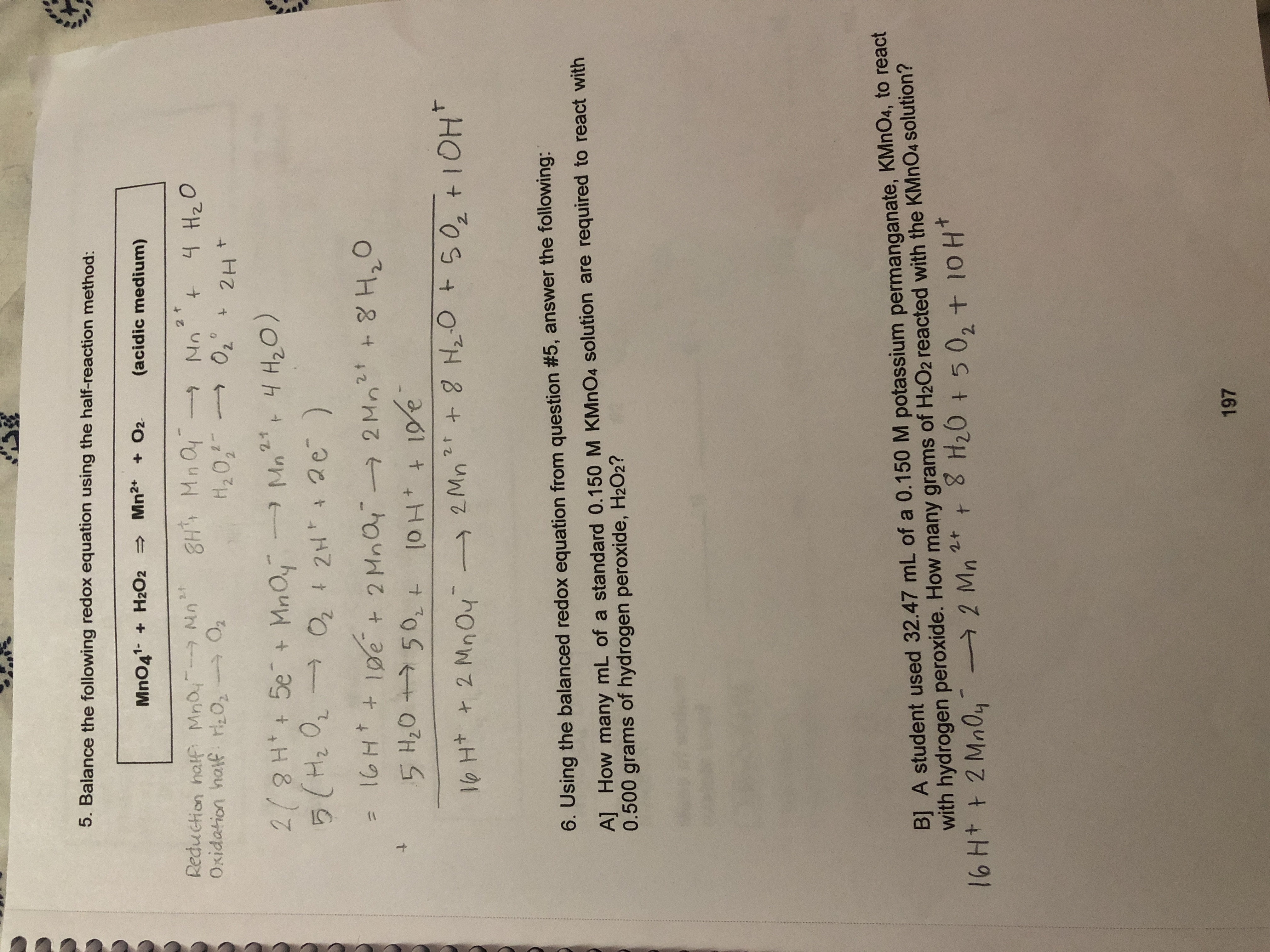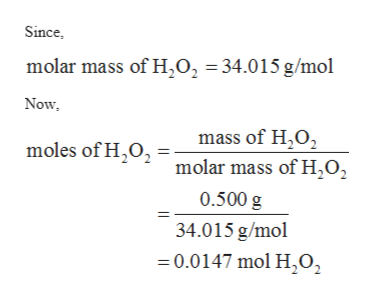# 5. Balance the following redox equation using the half-reaction method:MnO41 H2O2 Mn2 O2.(acidic medium)Reduction hatf Mno MOxidation hatf 20, O22t8H Mn aH2022 t4 Hz0No+0202Ht2/2H5e + MnOy5(H2 02.1tMn 4 H20)22H 2e)ipe+ 2 Mna2 Mn2 8 H,O5 H2O 50,t+ 8 HzO t50, + IOH2 tlo Ht t2 MnO4-2 Mn6. Using the balanced redox equation from question #5, answer the following:A] How many mL of a standard 0.150 M KMnO4 solution are required to react with0.500 grams of hydrogen peroxide, H2O2?B] A student used 32.47 mL of a 0.150 M potassium permanganate, KMnO4, to reactwith hydrogen peroxide. How many grams of H2O2 reacted with the KMNO4 solution?T6 H+ t 2 MnOy-8 H20 t502 + 10 H2tt2 Mn197T T(

Question
55 views

Question 6 Ahelp_outlineImage Transcriptionclose5. Balance the following redox equation using the half-reaction method: MnO41 H2O2 Mn2 O2. (acidic medium) Reduction hatf Mno M Oxidation hatf 20, O2 2t 8H Mn a H202 2 t 4 Hz0 No + 020 2Ht 2/2H5e + MnOy 5(H2 0 2.1 t Mn 4 H20) 22H 2e) ipe + 2 Mna 2 Mn2 8 H,O 5 H2O 50,t + 8 HzO t50, + IOH 2 t lo Ht t2 MnO4- 2 Mn 6. Using the balanced redox equation from question #5, answer the following: A] How many mL of a standard 0.150 M KMnO4 solution are required to react with 0.500 grams of hydrogen peroxide, H2O2? B] A student used 32.47 mL of a 0.150 M potassium permanganate, KMnO4, to react with hydrogen peroxide. How many grams of H2O2 reacted with the KMNO4 solution? T6 H+ t 2 MnOy- 8 H20 t502 + 10 H 2t t 2 Mn 197 T T ( fullscreen
check_circle

Step 1

The values are given as follows:

Step 2

The balanced chemical reaction can be written as follows:

Step 3

The number of moles of H2O2 can be calc...help_outlineImage TranscriptioncloseSince, molar mass of H202 =34.015 g/mol Now mass of H2O2 molar mass of H2O2 moles of H2O 0.500 g 34.015 g/mol =0.0147 mol H,0, fullscreen

### Want to see the full answer?

See Solution

#### Want to see this answer and more?

Solutions are written by subject experts who are available 24/7. Questions are typically answered within 1 hour.*

See Solution
*Response times may vary by subject and question.
Tagged in

### Physical Chemistry